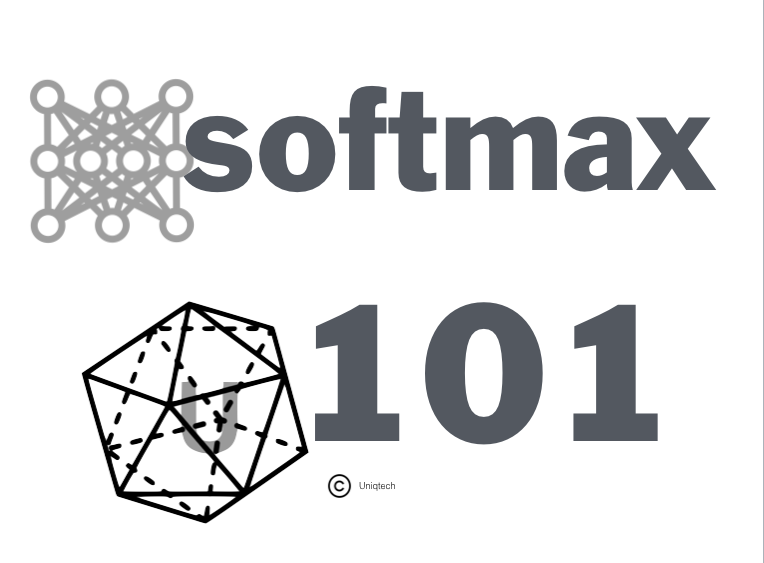# Understand the Softmax Function in MinutesUnderstanding Softmax in Minutes by Uniqtech

Learning machine learning? Specifically trying out neural networks for deep learning? You likely have run into the Softmax function, a wonderful activation function that turns numbers aka logits into probabilities that sum to one. Softmax function outputs a vector that represents the probability distributions of a list of potential outcomes. It’s also a core element used in deep learning classification tasks. We…

--

--